# Non Verbal Reasoning - Cubes and Dice

## Why Non Verbal Reasoning Cubes and Dice?

In this section you can learn and practice Non Verbal Reasoning Questions based on "Cubes and Dice" and improve your skills in order to face the interview, competitive examination and various entrance test (CAT, GATE, GRE, MAT, Bank Exam, Railway Exam etc.) with full confidence.

## Where can I get Non Verbal Reasoning Cubes and Dice questions and answers with explanation?

IndiaBIX provides you lots of fully solved Non Verbal Reasoning (Cubes and Dice) questions and answers with Explanation. Solved examples with detailed answer description, explanation are given and it would be easy to understand. All students, freshers can download Non Verbal Reasoning Cubes and Dice quiz questions with answers as PDF files and eBooks.

## Where can I get Non Verbal Reasoning Cubes and Dice Interview Questions and Answers (objective type, multiple choice)?

Here you can find objective type Non Verbal Reasoning Cubes and Dice questions and answers for interview and entrance examination. Multiple choice and true or false type questions are also provided.

## How to solve Non Verbal Reasoning Cubes and Dice problems?

You can easily solve all kind of Non Verbal Reasoning questions based on Cubes and Dice by practicing the objective type exercises given below, also get shortcut methods to solve Non Verbal Reasoning Cubes and Dice problems.

### Exercise :: Cubes and Dice - Section 1

The sheet of paper shown in the figure (X) given on the left hand side, in each problem, is folded to form a box. Choose from amongst the alternatives (1), (2), (3) and (4), the boxes that are similar to the box that will be formed.

1.

Choose the box that is similar to the box formed from the given sheet of paper (X).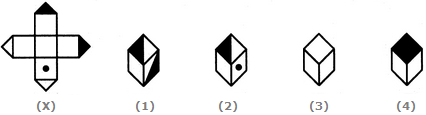A. 1 and 2 only B. 2 and 4 only C. 2 and 3 only D. 1 and 4 only

Explanation:

The fig. (X) is similar to the Form VI. So, when a cube is formed by folding the sheet shown in fig. (X), then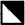is one of the faces of the cube. However, the cube in fig. (1) has two such faces and fig. (4) has a face which is completely shaded. So, these two cubes cannot be formed. Hence, only the cubes in figures (2) and (3) can be formed.

2.

Choose the box that is similar to the box formed from the given sheet of paper (X).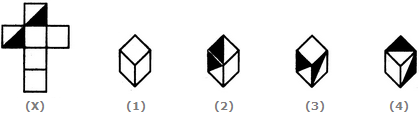A. 1 and 4 only B. 3 and 4 only C. 1 and 2 only D. 2 and 3 only

Explanation:

The fig. (X) is similar to the Form I. So, when the sheet shown in fig. (X) is folded to form a cube then one of the two half-shaded faces lies opposite to one of the blank faces and the other half-shaded face lies opposite to another blank face. The two remaining blank faces lie opposite to each other. Thus, both the cubes shown in figures (1).and (4) can be formed when the sheet shown in fig. (X) is folded. Also, though the cubes shown in figures (2) and (3) have faces that can appear adjacent to each other but the cube formed by folding the sheet in fig. (X) cannot be rotated to form either of the two. Hence, the cubes in figures (2) and (3) cannot be formed.

3.

How many dots lie opposite to the face having three dots, when the given figure is folded to form a cube?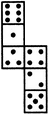A. 2 B. 4 C. 5 D. 6

Explanation:

The given figure is similar to Form V. Therefore, when this figure is folded to form a cube then the face bearing six dots will lie opposite the face bearing three dots.

4.

Choose the box that is similar to the box formed from the given sheet of paper (X).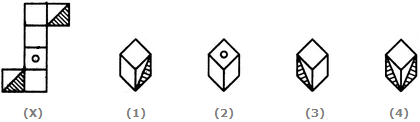A. 1 and 3 only B. 1 and 4 only C. 2 and 4 only D. 3 and 4 only

Explanation:

The fig. (X) is similar to Form II. So, when the sheet shown in fig. (X) is folded to form a cube then the two half-shaded faces lie opposite to each other, the face bearing a circle lies opposite to one of the two blank faces and the two remaining blank faces lie opposite to each other. Therefore, the cubes shown in fig. (4) which has the two half-shaded faces adjacent to each other, cannot be formed by folding the sheet shown in fig. (X). Also, the cube shown in fig. (2) has the face bearing a circle adjacent to two blank faces. This is not possible since there is one blank face opposite to the circle and one blank face opposite to the third blank face. Hence, only the cubes in figures (1) and (3) can be formed.

5.

Choose the box that is similar to the box formed from the given sheet of paper (X).A. 1 and 2 only B. 2 and 3 only C. 2 and 4 only D. 1, 2, 3 and 4